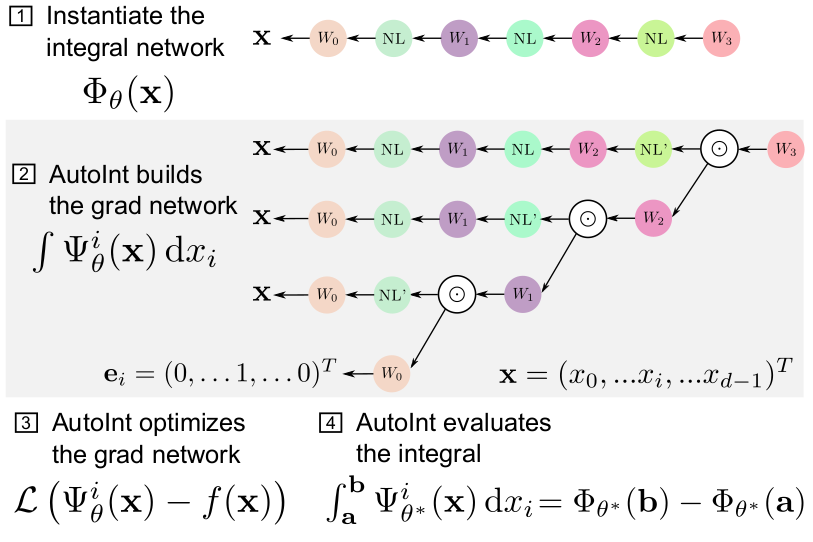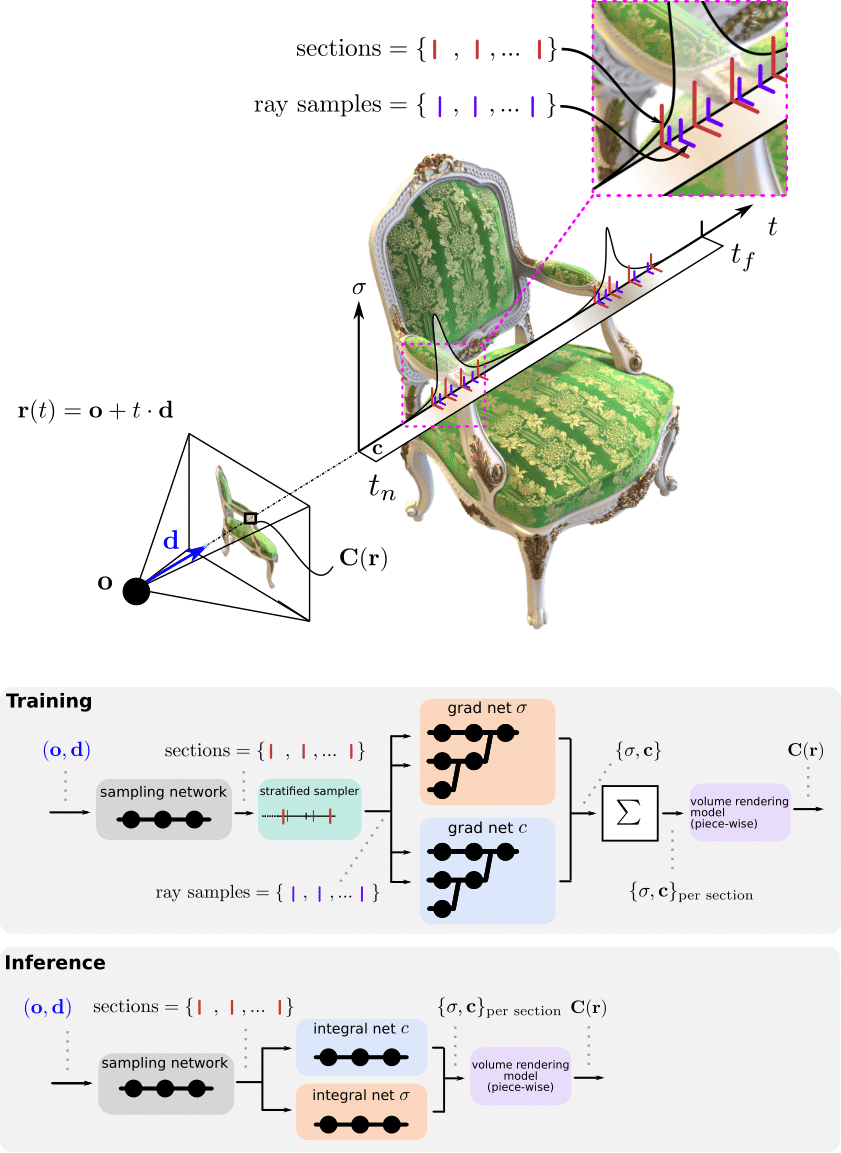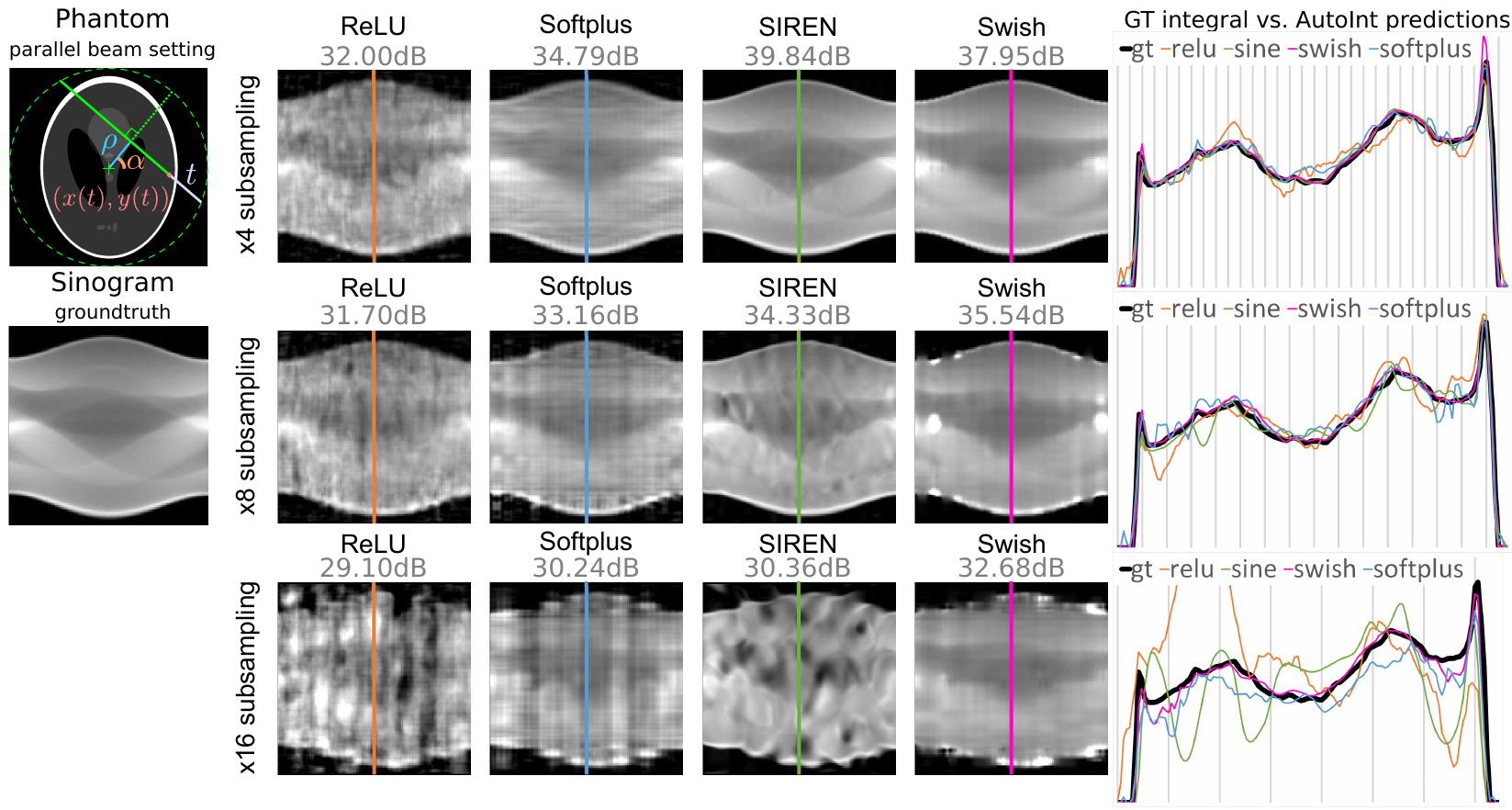# AutoInt: Automatic Integration for Fast Neural Volume Rendering

### Abstract

Numerical integration is a foundational technique in scientific computing and is at the core of many computer vision applications. Among these applications, neural volume rendering has recently been proposed as a new paradigm for view synthesis, achieving photorealistic image quality. However, a fundamental obstacle to making these methods practical is the extreme computational and memory requirements caused by the required volume integrations along the rendered rays during training and inference. Millions of rays, each requiring hundreds of forward passes through a neural network are needed to approximate those integrations with Monte Carlo sampling. Here, we propose automatic integration, a new framework for learning efficient, closed-form solutions to integrals using coordinate-based neural networks. For training, we instantiate the computational graph corresponding to the derivative of the coordinate-based network. The graph is fitted to the signal to integrate. After optimization, we reassemble the graph to obtain a network that represents the antiderivative. By the fundamental theorem of calculus, this enables the calculation of any definite integral in two evaluations of the network. Applying this approach to neural rendering, we improve a tradeoff between rendering speed and image quality: improving render times by greater than 10× with a tradeoff of slightly reduced image quality.

Publication
IEEE/CVF Computer Vision and Pattern Recognition (CVPR 2021)

## The AutoInt Framework

### Principle

After (1) defining an integral network architecture, (2) AutoInt builds the corresponding grad network, which is (3) optimized to represent a function. (4) Definite integrals can then be computed by evaluating the integral network, which shares parameters with its grad network.### Volume rendering pipeline

During training, the grad networks representing volume density σ and color c are optimized for a given set of multi-view images (top left). For inference, the grad networks’ parameters are reassembled to form the integral networks, which represent antiderivatives that can be efficiently evaluated to calculate ray integrals through the volume (bottom left). A sampling network predicts the locations of piecewise sections used for evaluating the definite integrals (right).## Results

### Sparse View Computed Tomography

Left: illustration of the parameterization. Center: sinograms computed with the integral networks using different nonlinear activation functions. The ground truth (GT) sinogram is subsampled in angle by 4× (top), 8× (middle), and 16× (bottom). The optimized networks are used to interpolate the missing measurements. Using the Swish activation performs best in these experiments. Right: 1D scanlines of the sinogram centers shows the interpolation behavior of each method for each subsampling level.